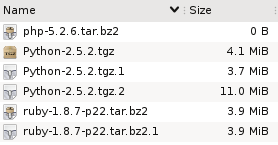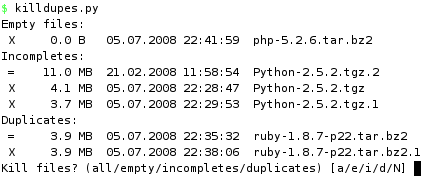## killdupes: detect duplicate and incomplete filesJuly 6th, 2008Suppose you have these files in a directory. What do you think has happened here?

Well, it looks like someone tried to download the php interpreter, and for some reason that didn't work, because the file is empty.

Then we have two copies of the ruby distribution, and both have the same size. So it looks like that download did complete successfully, but maybe the person downloaded it once and then forgot about it?

Finally, there are three files that contain the python distribution, but they are all different sizes. Since we know that `wget` adds extensions like `.1` to avoid overwriting files, it looks like the first two attempts at this download failed, and the third succeeded.

### Three types of redundant files

These three cases demonstrate classes of redundant files:

1. Empty files.
The filename hints at what the intended content was, but since there is no data, there's no way to tell.
2. Duplicate files.
Multiple files have the exact same content.
3. Incomplete/partial files.
The content of one file comprises the partial content of another, larger file. The smaller file is a failed attempt at producing the larger one.

Now look back at the first screenshot and tell me how quickly would you detect these three cases if a) the files didn't have descriptive names (random names, say), and b) they were buried in a directory of 1000 other files? More importantly, how long would you even bother looking?What if you had a tree of directories where you wanted to eliminated duplicate or incomplete files? Even worse.

### How to proceed?

Finding empty files is trivial. Finding duplicate files is also relatively easy if you just look for files that have the same size. But to be sure that they are equal, you have to actually examine their contents. One way is to compute checksums (`md5`, for instance) and compare them.

But that doesn't help with partial files, because any file that is smaller than another file could potentially be incomplete relative to the larger one.

I set out to solve this without expecting that it would be very hard, but it turns out to be complicated. The code is rather ugly and I wonder if there is an easier way. In a nutshell, we read sequential chunks from all the files in the directory, hashing the data as we go along. The hashes then become keys in a dictionary, which will put files whose chunks hash to the same value in the same bucket. And that's how we know they are identical. As long as any bucket has more than two files (ie. so far they are the same), we keep on going.

All in all, we keep reading chunks from those files (beginning with all) whose data produce a hash that belongs to more than one file. In other words, we have to read all the common bytes in all the files + one extra chunk from each (to determine that the commonality has ended).

The size of a chunk is set to 100kb, but will be determined by how far we can read into a file depending on the size of the other files "in the running". Suppose we are at position (offset) 100kb, where one file is 300kb and another is 110kb, then we can only read 10kb from each to check if the data is the same. Whatever the outcome, we don't need to read any more than that, because we've reached the end of the smaller one.

Obviously, this won't work on files that aren't constructed sequentially (torrent and whatever).

### The nitty gritty

The center piece of the code is a hash table called `offsets`. The keys, indeed, represent offsets into the mass of files we are working on. The values are themselves hash tables which contain hashes of chunks read from the files.

So we start out in `offsets`, which is a hash table with only one item, containing all of the files. We read up to 100kb from each file, until we reach the chunk size or the end of the smallest file. The size read is captured in `readsize`, which determines `new_offset`. We will now have a new entry called `offsets[new_offset]`. We how hash (with md5) a `readsize` number of bytes of data from each file, so that each hash becomes an entry into the new hash table at `offsets[new_offset]`. As the value we store the file that produced it.

And so it continues. Every iteration produces a new offset and a new set of hashes, in each case the new hash combines the old hash with the new data. In the end, we have a number of lists indexed by hash at every offset level. If there are multiple files in such a list, then they have all hashed to the same value up to this point in the file (they are the same so far).

The interesting lists are those where at least one file has reached eof. Because that means we have read the whole file and its full contents is equal to that of another file. If they are the same size (both have reached eof), they are duplicates of each other. If not, the smaller is incomplete relative to the larger.

### How lazy are we?

Unfortunately, it's hard to predict how many bytes have to be read, because that depends entirely on how much the files have in common. If you have two copies of an 700mb iso image you might as well `md5sum` them both, we can't do any better than that. But if they aren't the same, we stop reading chunks as soon as they become distinct, which is likely to be at the very beginning. Equally, memory use will be highest at the start since we're reading from all of the files.

### Performance

The slowest part of execution is obviously disk io. So what if we run it on a hot cache so that all (or most) of the blocks are already in memory?

In the worst case, we have two 700mb files that are identical (populated from `/dev/urandom`).

5.6s  md5sum testfile*
9.4s  killdupes.py 'testfile*'

Slower, but not horribly slow. Now let's try the best case. Same files, but we delete the first byte from one of them, displacing every byte by one to the left relative to the original.

6.2s   md5sum testfile*
0.02s  killdupes.py 'testfile*'

And there it is! No need to hash the whole thing since they're not equal, not even at byte 1.

On the other hand, a rougher test is to set it free on a directory with lots of files. For instance, in a directory of 85k files, there are 42 empty files, 7 incomplete and 28 duplicates. We had to read 2gb of data to find all these. Not surprisingly, md5sum didn't enjoy that one as much.

41m  md5sum
36m  killdupes.py

So that's pretty slow, but can you make it faster?

```#!/usr/bin/env python
#
# Author: Martin Matusiak <numerodix@gmail.com>
# Licensed under the GNU Public License, version 3.
#
# revision 3 - Sort by smallest size before reading files in bucket
# revision 2 - Add dashboard display
# revision 1 - Add total byte count

from __future__ import with_statement
import glob
import hashlib
import os
import sys
import time

CHUNK = 1024*100
BYTES_READ = 0

_units = { 0: "B", 1: "KB", 2: "MB", 3: "GB", 4: "TB", 5: "PB", 6: "EB"}

class Record(object):
def __init__(self, filename, data=None, eof=False):
self.filename = filename
self.data = data
self.eof = eof

def format_size(size):
if size == None:
size = -1

c = 0
while size > 999:
size = size / 1024.
c += 1
r = "%3.1f" % size
u = "%s" % _units[c]
return r.rjust(5) + " " + u.ljust(2)

def format_date(date):
return time.strftime("%d.%m.%Y %H:%M:%S", time.gmtime(date))

def format_file(filename):
st = os.stat(filename)
return ("%s  %s  %s" %
(format_size(st.st_size), format_date(st.st_mtime), filename))

def write(s):
sys.stdout.write(s)
sys.stdout.flush()

def clear():
write(79*" "+"\r")

def write_fileline(prefix, filename):
write("%s %s\n" % (prefix, format_file(filename)))

def get_hash(idx, data):
m = hashlib.md5()
m.update(str(idx) + data)
return m.hexdigest()

def get_filelist(pattern=None, lst=None):
files = []
it = lst or glob.iglob(pattern)
for file in it:
file = file.strip()
if os.path.isfile(file) and not os.path.islink(file):
files.append(Record(file))
return files

def get_chunk(offset, length, filename):
try:
with open(filename, 'r') as f:
f.seek(max(offset,0))
data = f.read(length)
ln = len(data)
global BYTES_READ
BYTES_READ += ln
return ln, data
except IOError, e:
write("%s\n" % e)
return 0, ""

def short_name(lst):
lst.sort(cmp=lambda x, y: cmp((len(x), x), (len(y), y)))
return lst

def rev_file_size(lst):
lst.sort(reverse=True,
cmp=lambda x, y: cmp(os.path.getsize(x), os.path.getsize(y)))
return lst

def rec_file_size(lst):
lst.sort(cmp=lambda x, y: cmp(os.path.getsize(x.filename),
os.path.getsize(y.filename)))
return lst

def compute(pattern=None, lst=None):
zerosized = []
incompletes = {}
duplicates = {}

offsets = {}
offsets = {}
key = get_hash(0, "")

write("Building file list..\r")
offsets[key] = get_filelist(pattern=pattern, lst=lst)

offsets_keys = offsets.keys()
for offset in offsets_keys:
offset_hashes = [(h,r) for (h,r) in offsets[offset].items() if len(r) > 1]
buckets = len(offset_hashes)
for (hid, (hash, rs)) in enumerate(offset_hashes):
rs = rec_file_size(rs) # sort by shortest to not read redundant data
reads = []
readsize = CHUNK
for (rid, record) in enumerate(rs):
ln, data = get_chunk(offset, readsize, record.filename)
s = ("%s | Offs %s | Buck %s/%s | File %s/%s | Rs %s" %
(format_size(BYTES_READ),
format_size(offset),
hid+1,
buckets,
rid+1,
len(rs),
format_size(readsize)
)).ljust(79)
write("%s\r" % s)
if ln == 0:
record.eof = True
else:
r = Record(record.filename, data)
if ln < readsize:
readsize = ln
reads.append(r)

if reads:
new_offset = offset+readsize
if new_offset not in offsets:
offsets[new_offset] = {}
offsets_keys.append(new_offset)
offsets_keys.sort()

for r in reads:
new_hash = get_hash(new_offset, hash+r.data[:readsize])
r.data = None
if new_hash not in offsets[new_offset]:
offsets[new_offset][new_hash] = []
offsets[new_offset][new_hash].append(r)
clear() # terminate offset output

offsets_keys = offsets.keys()
offsets_keys.sort(reverse=True)
for offset in offsets_keys:
offset_hashes = offsets[offset]
for (hash, rs) in offset_hashes.items():
if offset == 0:
zerosized = [r.filename for r in rs if r.eof]
else:
if len(rs) > 1:
eofs = [r for r in rs if r.eof]
n_eofs = [r for r in rs if not r.eof]
if len(eofs) >= 2 and len(n_eofs) == 0:
duplicates[eofs.filename] = [r.filename for r in eofs[1:]]
if len(eofs) >= 1 and len(n_eofs) >= 1:
key = rev_file_size([r.filename for r in n_eofs])
if not key in incompletes:
incompletes[key] = []
for r in eofs:
if r.filename not in incompletes[key]:
incompletes[key].append(r.filename)

return zerosized, incompletes, duplicates

def main(pattern=None, lst=None):
zerosized, incompletes, duplicates = compute(pattern=pattern, lst=lst)
if zerosized or incompletes or duplicates:

kill = " X "
keep = " = "

q_zero = []
q_inc  = []
q_dupe = []

if zerosized:
write("Empty files:\n")
for f in zerosized:
q_zero.append(f)
write_fileline(kill, f)

if incompletes:
write("Incompletes:\n")
for (idx, (f, fs)) in enumerate(incompletes.items()):
fs.append(f)
fs = rev_file_size(fs)
for (i, f) in enumerate(fs):
prefix = keep
if os.path.getsize(f) < os.path.getsize(fs):
q_inc.append(f)
prefix = kill
write_fileline(prefix, f)
if idx < len(incompletes) - 1:
write('\n')

if duplicates:
write("Duplicates:\n")
for (idx, (f, fs)) in enumerate(duplicates.items()):
fs.append(f)
fs = short_name(fs)
for (i, f) in enumerate(fs):
prefix = keep
if i > 0:
q_dupe.append(f)
prefix = kill
write_fileline(prefix, f)
if idx < len(duplicates) - 1:
write('\n')

inp = raw_input("Kill files? (all/empty/incompletes/duplicates) [a/e/i/d/N] ")

if "e" in inp or "a" in inp:
for f in q_zero: os.unlink(f)
if "i" in inp or "a" in inp:
for f in q_inc: os.unlink(f)
if "d" in inp or "a" in inp:
for f in q_dupe: os.unlink(f)

if __name__ == "__main__":
pat = '*'
if len(sys.argv) > 1:
if sys.argv == "-h":
write("Usage:  %s ['<glob pattern>'|--file <file>]\n" %
os.path.basename(sys.argv))
sys.exit(2)
elif sys.argv == "--file":
lst = open(sys.argv, 'r').readlines()
main(lst=lst)
else:
pat = sys.argv
main(pattern=pat)
else:
main(pattern='*')
```

:: random entries in this category ::